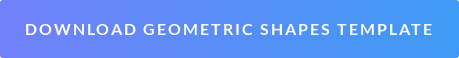# Free Geometric Shapes Learning Template! 2D and 3D Shapes That You Need to Know

•## Contents

Cubes and cuboids, prisms and pyramids- What does it all mean?

We’re here to break geometry down for you in our introduction to geometry. We’ve made these handy summary sheets to help you to differentiate your 2D from 3D shapes and we’ll go into more detail so that you’ll be sure to know your parallelograms from your pentagons.## What is geometry?

Geometry is a topic in maths that is all about shapes and their properties.

We’ll look closely at 2D shapes (flat shapes) and 3D shapes (with height, width and depth) so you’ll know your cylinder from your trapeze in no time!

Geometry is great because unlike more abstract topics in maths we can see many examples of geometry in our everyday lives.

We are not dealing with just numbers but real solid shapes that exist around us. I’m sure that you can spot at least three different shapes from where you are right now- that’s geometry!

## Why is geometry important?

There are loads of real-world uses for geometry so it’s important to take some time to get your head around it. Geometry also directly links to other areas of maths such as trigonometry and algebra. So having a solid knowledge of geometry is important to succeed in maths.

What are some uses for geometry?

Geometry is everywhere in both the man-made and natural world.

• Geometric shapes are the basis for creating immersive worlds in video games
• Architecture also uses geometry, since buildings are constructed from 3D shapes that fit together.
• Art is based on geometry, as shapes are used to create images and patterns in a painting and the formation and combination of different 3D shapes come together to create a sculpture.

If you want to practice at home, click the button below to download our free cut and stick geometric shapes template!## Where can you find geometry in the natural world?

Perfectly formed geometrical shapes exist everywhere in nature if we take a moment to look.

• The hexagon cells inside a beehive.
• The Earth is a sphere.
• A pinecone is shaped like a cone.

## The most important geometric 2D shapes

You probably know some of these already, but let’s recap them to be sure:

• Rectangle- Four straight sides and four right angles, opposite sides are the same length and one pair of sides is longer than the other pair.
• Square- Four straight sides and four right angles, all sides are the same length.
• Quadrilateral- Four sides. Rectangles, squares, rhombuses, and trapezes are all quadrilaterals!
• Triangles- Three straight sides and three angles (we’ll discuss the specific types separately).
• Trapeze- Four straight sides and one pair of parallel sides.
• Parallelogram- A type of quadrilateral with two pairs of parallel sides and no right angles.
• Rhombus- A type of parallelogram with four sides (not straight) and four angles (not right angles), equal opposite sides and equal opposite angles. The diagonals bisect each other.
• Pentagon- Five sides and five equal angles.
• Hexagon- Six sides and six equal angles.
• Octagon- Eight sides and eight equal angles.

## So what is the difference between parallelograms and quadrilaterals?

All parallelograms are quadrilaterals, but not all quadrilaterals are parallelograms! A quadrilateral is any shape with four sides, a parallelogram is more specific because while it must have four sides, there are no right angles and there are two pairs of parallel sides.

Triangles are explored even more in another branch of maths called ‘Trigonometry’ but we touch on them here in geometry too so let's take a look at some of the different triangles.

• Isosceles triangle- Two sides are equal or congruent.
• Equilateral triangle- All three sides have the same length (they are ‘equal’ which sounds a bit like equilateral).
• Scalene triangle- None of the angles are equal.
• Acute triangle-  All angles measure less than 90°.
• Right angle triangle- One angle is 90°.
• Obtuse triangle-  One angle measures more than 90°.

## The most important geometric 3D shapes

• Cuboid- Six rectangular faces at right angles to each other.
• Cube- Six equal squares at right angles to each other.
• Prism- Two identical shapes facing each other, the other faces are quadrilaterals.
• Pyramid- A square or triangle-based shape, all other sides are triangles that meet at a point.
• Cone- Circular base with one rounded edge that meets at a point.
• Sphere- One faced, rounded and like a ball.
• Cylinder- Two circular faces that are parallel and one curved rectangular shape between the circular faces.

## Did you know this about geometric shapes?

• Euclid is called the father of geometry, he lived in ancient Greece. But, it’s hard to pin down a single founder as Euclid’s works were likely built on others that might have been lost through history.
• It is thought that geometry was first discovered to help with construction and astronomy.
• Geometry and algebra are both very common branches of maths, but it’s believed that geometry was developed 1100 years after algebra.

Getting in a muddle with geometry? Why not have a free trial lesson with one of our GoStudent geometry tutors who can break it down for you?